Blog

# 5 Ways To Get Students Inspired About the Wave Model Of Light

Grace Chukwuekwu
Teaching with Labster
October 25, 2022

Electromagnetic waves, or EM waves, are waves generated from oscillations between electric and magnetic fields. Visible light waves are part of electromagnetic waves. Light exhibits certain behaviors that cannot be explained by considering light as a particle. These behaviors include reflection and refraction, but also diffraction, interference, and the Doppler effect, all wave behavior.

Wave-particle duality is the concept that particles can behave like waves and waves can behave like particles. For example, light can be described and studied as light waves and/or photon particles.

There are several reasons why students are afraid of the wave model of light. We've also listed some practical solutions teachers can use in future classes to make theory and practice easier for their students. Finally, we share why virtual lab simulations are beneficial not only for your students but also for you as an educator to convey concepts more effectively.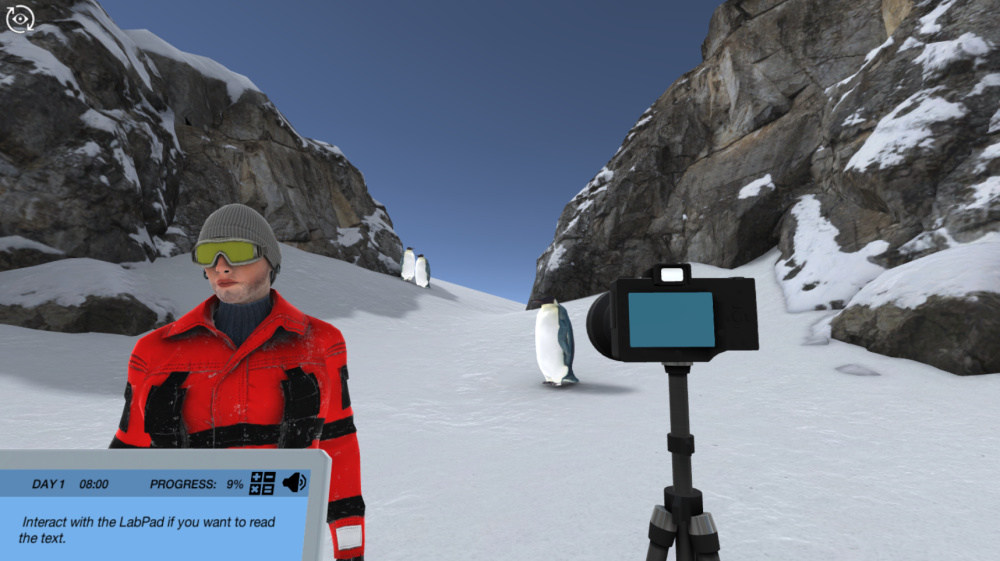Figure 1: An image showing a photograph-taking process during a visit to Antarctica is available in the wave model of light simulation from Labster. The simulation is usable for High School and University/College courses.

## Why the Wave Model Of Light  Can Be Tricky For Student To Grasp

There are three reasons In particular, wave models of light can be difficult, even for the most energetic of students.

### 1. It feels abstract

The wave model describes how light propagates like we model ocean waves moving through water. By imagining light as an oscillating wave, we can explain the properties of light, such as its wavelength and frequency, but these are not visible to students so they see this topic as just imaginary and difficult to understand.

### 2. It’s content-heavy

The photoelectric effect is the emission of electrons from materials, especially metals when electromagnetic waves (e.g., light) of a certain frequency - frequencies greater than a threshold value - strike their surface. If electromagnetic radiation has a frequency below the threshold, the electrons cannot gain enough energy to overcome the electrostatic barrier of the metalwork function. Each type of surface and material has a unique threshold.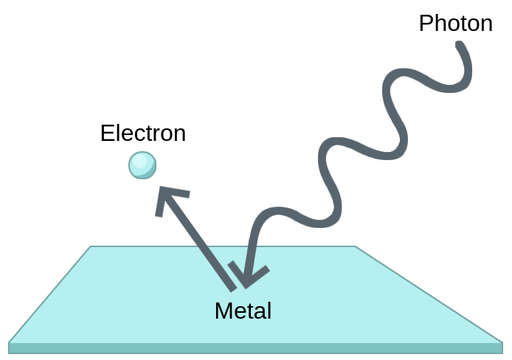Figure 2: A simple representation of the photoelectric effect.

Photons are light particles, meaning they are quantifiable objects that carry a measurable amount of electromagnetic energy. Photons travel at the speed of light and are usually thought of as particles with no mass and no electric charge. Photons are now considered as particles in the field concerned with the interaction of materials with absorbed and emitted light, and as waves in the scientific field concerned with the propagation of light.

### 3. When facts are not explained to students

The speed of light depends on the frequency and wavelength as described in the equation below:

c = λf

where c is the speed of light (measured in meters per second), f is the frequency of light in Hertz (Hz) and is the wavelength of light, measured in meters. This equation also applies to other types of electromagnetic waves. From this equation, it can be concluded that an increase in frequency causes a decrease in the wavelength of light. The photons that make up light also have a higher energy at higher frequencies.

Electromagnetic Waves

Electromagnetic waves are characterized by a variety of wavelengths and frequencies, each associated with a specific intensity (or amplitude) and amount of energy. This wide range of wavelengths describes the electromagnetic spectrum. Electromagnetic waves propagate (move) in the direction of the oscillating vector of electric and magnetic oscillating fields. The two fields are perpendicular to each other and transport energy from the radiation source to an undetermined final destination. Like mechanical waves, each electromagnetic wave oscillates periodically with peaks and valleys and has a characteristic amplitude, wavelength, and frequency.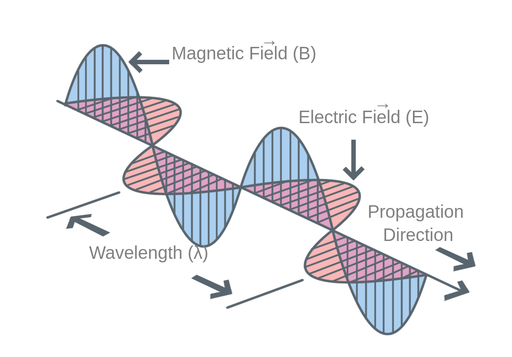Figure 3: The electric and magnetic oscillating field vectors.

Amplitude refers to the magnitude of the difference between the maximum position of a particle or wave compared to its resting position.

The wavelength is the length of one complete wave cycle. In simple harmonic motion, after reaching a distance of one wavelength, the wave begins to repeat itself.

## 5 Ways To Make Wave Model Of Light A More Approachable Topic

With these points in mind, here are five things you can consider introducing into your wave model of light lessons to make them more engaging, approachable, and enjoyable to teach for you and to learn for your students.

### 1. Show the people behind the science

Providing a brief history of how the concept of visible light behaves as a particle and as a wave will stimulate students to become more interested in the wave model of light

In 1905, Albert Einstein published his theory of the photoelectric effect, which stated that light can also behave like particles. This theory was extended in 1924 by Louis de Broglie, a French physicist, who suggested that electrons and all matter could have wave properties. This complementary hypothesis has since been tested and proven correct, leading to the current assumption that wave and particle properties can overlap.

### 2. Define terms clearly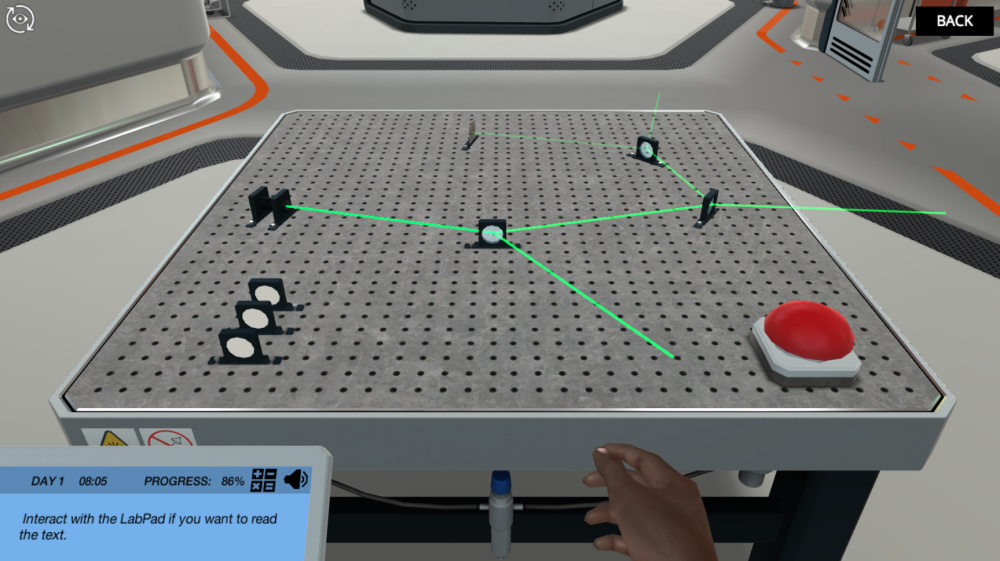Figure 4:  An image from a wave model of light simulation from Labster. The simulation is usable for High School and University/College courses.

Defining basic terminology related to visible light makes it easier to understand how the wave model of light works. This makes it easier for teachers to guide students, and in turn, students can see it as real. Below are the terminologies related to visible light as an electromagnetic wave:

• The frequency f is proportional to the reciprocal of the wavelength and is related to the speed of light (c) in a vacuum. This means that longer wavelengths correspond to lower frequencies and shorter wavelengths to higher frequencies. A second definition is that frequency is also defined as the number of complete cycles occurring per period, T, of time. The SI unit of frequency is Hertz, defined as 1 Hz = 1 s-1. The shorter the period, the larger the frequency. The following equation defines frequency as the reciprocal of the period of the oscillation, T.

• The electromagnetic spectrum is a continuum of all electromagnetic waves arranged according to their wavelength, and frequency. Types of electromagnetic waves that make up the electromagnetic spectrum are radio waves, microwaves, infrared light, visible light, ultraviolet light, X-rays, and gamma rays. The shortest waves are gamma rays, which have wavelengths of 10e-6 microns or less. The longest waves are radio waves, which can have wavelengths of many kilometers. The range of visible electromagnetic waves consists of the narrow section between 390nm (blue) to 700nm (red).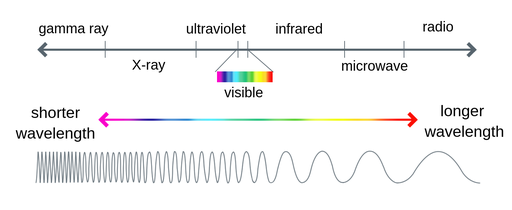• Reflection involves changing the direction of the waves as they bounce off an obstacle. Reflection can be visualized as a beam of light hitting a mirror.

• Refraction of waves involves changing the direction of waves as they pass from one medium to another. Rays coming from the air through the water cause changes in the direction and intensity of the light. The path of a light wave is curved as it crosses the boundary between two media. This phenomenon is caused by the change in velocity that the wave undergoes as it changes the medium, and the angle of refraction is governed by Snell's law.

### 3. Seeing is believing

Total internal reflection describes the total internal reflection of a ray of light that passes through a dense medium and reaches the boundary with a less dense medium. This only happens when the angle of incidence exceeds the critical angle. The critical angle is the greatest angle of incidence (when a ray of light reaches the boundary between two media with different intensities) at which refraction can still occur. For every angle of incidence that is greater than the critical angle, the ray undergoes total internal reflection.

The basic law governing the refraction of light is Snell's law. According to Snell's law, when a beam of light is passed into a new medium, the relationship between the angle of incidence (θi) and the angle of refraction (θr) is given by the following equation

ni sin(θi) = nr sin(θr)

where the ni and nr values represent the refractive indexes (which are unitless) of the incident and the refractive medium, respectively.

### 4. Make it stick with word-play

Memory aids can be very helpful in understanding complex topics such as wave model of light and can be used in recalling the types of electromagnetic waves that make up the electromagnetic spectrum using "GIVe X RUM" as a memory aid and represents the following:

G - Gamma rays

I - Infrared light

V - Visible light

X - X-rays

U - Ultraviolet light

M - Microwaves

### 5. Encourage the use of virtual lab simulations

A unique way to teach wave models of light is through a virtual laboratory simulation. At Labster, we’re dedicated to delivering fully interactive advanced laboratory simulations that utilize gamification elements like storytelling and scoring systems, inside an immersive and engaging 3D universe.

Check out the Labster wave model of light simulation that allows students to learn through active, inquiry-based learning. In the simulation, students will learn how reflection and refraction of light affect the photos you take among the snow and ice.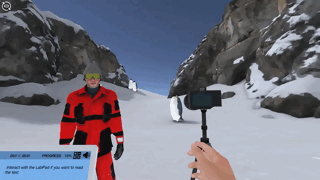Learn more about the wave model of light simulation here or get in touch to find out how you can start using virtual labs with your students.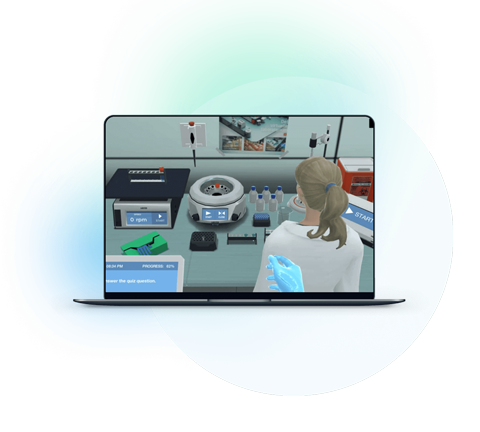What exactly are virtual labs?